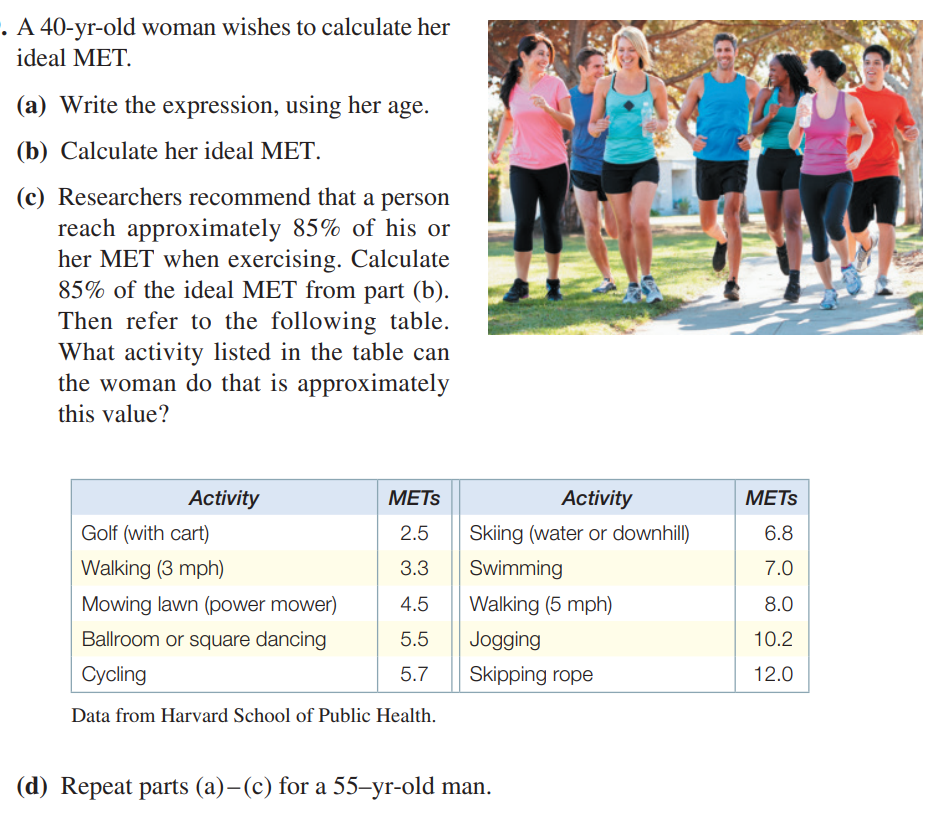### ¿Todavía tienes preguntas de matemáticas?

Pregunte a nuestros tutores expertos
Algebra
PreguntaA 40-yr-old woman wishes to calculate her ideal MET.

(a) Write the expression, using her age.

(b) Calculate her ideal MET.

(c) Researchers recommend that a person reach approximately $$85 \%$$ of his or her MET when exercising. Calculate $$85 \%$$ of the ideal MET from part (b). Then refer to the following table. What activity listed in the table can the woman do that is approximately this value?

(d) Repeat parts (a)-(c) for a 55-yr-old man.

$$\left. \begin{array} { l } { \text { 109. (a) } 14.7 - 40 \cdot 0.13 \quad \text { (b) } 9.5 \quad \text { (c) } 8.075 ; \text { walking } ( 5 mph ) } \\ { \text { (d) } 14.7 - 55 \cdot 0.11 ; 8.65 ; 7.3525 \text { , swimming } \text { 111. Alaska, Texas, } } \end{array} \right.$$